Positive-definite kernel

(diff) ← Older revision | Latest revision (diff) | Newer revision → (diff)
A complex-valued functionon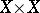, whereis any set, which satisfies the condition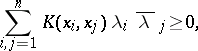for any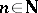,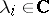,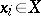. The measurable positive-definite kernels on a measure spacecorrespond to the positive integral operators (cf. Integral operator) on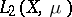; in order to include arbitrary positive operators in this correspondence one has to introduce generalized positive-definite kernels, which are associated with Hilbert spaces .
The theory of positive-definite kernels extends the theory of positive-definite functions (cf. Positive-definite function) on groups: For a functionon a groupto be positive definite it is necessary and sufficient that the function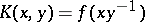onis a positive-definite kernel. In particular, certain results from the theory of positive-definite functions can be extended to positive-definite kernels. For example, Bochner's theorem is that each positive-definite function is the Fourier transform of a positive bounded measure (i.e. an integral linear combination of characters), and this is generalized as follows: Each (generalized) positive-definite kernel has an integral representation by means of so-called elementary positive-definite kernels with respect to a given differential expression .